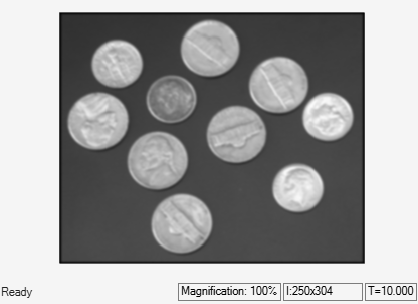# Blur Image Using Gaussian Kernel

This example shows you how to perform 2-D convolution to blur an image using the Gaussian kernel.

### Example Model

```modelname = "ex_blk2DConvolution.slx"; open_system(modelname); ```This model reads a PNG image using the Image From File block, which outputs it as a matrix of data type `double`.

The model then blurs the image by using a 2-D Convolution block to convolve the input image with a 7-by-7 representation of the Gaussian kernel.

### Simulate the Model

Run the model. The model displays the input image and the blurred output image using Video Viewer blocks.

```sim(modelname); ```